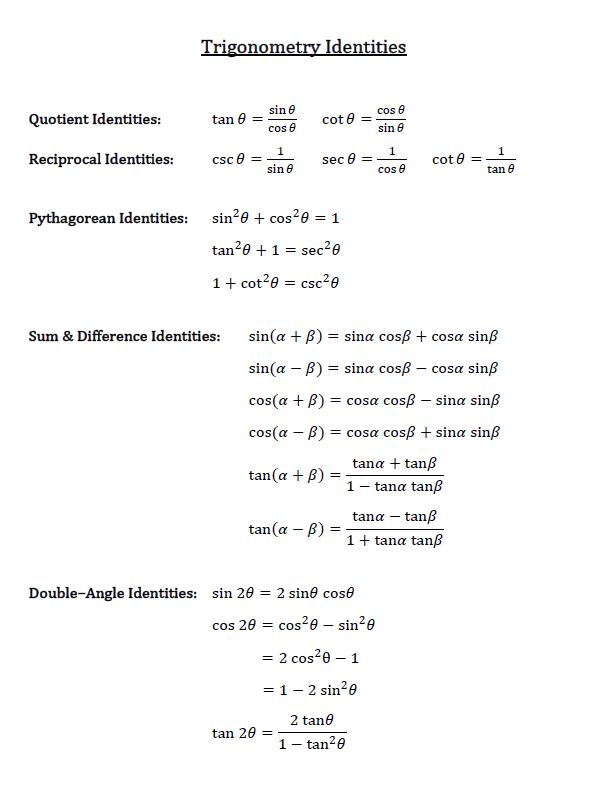# Sum and difference identities

0/8
##### Examples
###### Lessons
1. Simplify expressions
1. sin 24°cos 36° + cos 24°sin 36°
2. $\frac{tan {2 \pi \over 5 } - tan {3 \pi \over 20}}{1 + \tan {2 \pi \over 5} \cdot \tan {3 \pi \over 20}}$
2. Prove Identities
1. $\frac{\sin (A - B)}{\sin B} + \frac{\cos (A - B)}{\cos B} = \frac{\sin A}{\sin B \cos B}$
2. $\frac{1 + \tan A}{\tan (A + {\pi \over 4})} = 1 - \tan A$
3. Without using a calculator, evaluate:
1. sin 15°
2. sec (-105°)
3. tan ${19\pi \over 12}$
4. Given $\sin A = -{4 \over5}$ and $\cos B = {12 \over 13}$,
where $\pi \leq A \leq {3 \pi \over 2}$ and ${3 \pi \over 2} \leq B \leq 2\pi$,
find the exact value of $\cos (A + B)$
###### Free to Join!
StudyPug is a learning help platform covering math and science from grade 4 all the way to second year university. Our video tutorials, unlimited practice problems, and step-by-step explanations provide you or your child with all the help you need to master concepts. On top of that, it's fun - with achievements, customizable avatars, and awards to keep you motivated.
• #### Easily See Your ProgressWe track the progress you've made on a topic so you know what you've done. From the course view you can easily see what topics have what and the progress you've made on them. Fill the rings to completely master that section or mouse over the icon to see more details.
• #### Make Use of Our Learning Aids###### Practice Accuracy

See how well your practice sessions are going over time.

Stay on track with our daily recommendations.

• #### Earn Achievements as You LearnMake the most of your time as you use StudyPug to help you achieve your goals. Earn fun little badges the more you watch, practice, and use our service.
• #### Create and Customize Your AvatarPlay with our fun little avatar builder to create and customize your own avatar on StudyPug. Choose your face, eye colour, hair colour and style, and background. Unlock more options the more you use StudyPug.
###### Topic Notes
Trig identities are formulas developed based on Pythagorean Theorem. These identities show us how and where to find the sine, cosine, and tangent of the sum and difference of two given angles.$\sin (A + B)$
$\sin (A - B)$
$\cos (A + B)$
$\cos (A - B)$
$\tan (A + B)$
$\tan (A - B)$